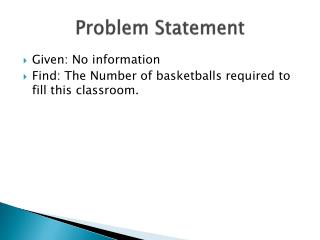DownloadDownload PresentationProblem Statement

# Problem Statement

Télécharger la présentation## Problem Statement

- - - - - - - - - - - - - - - - - - - - - - - - - - - E N D - - - - - - - - - - - - - - - - - - - - - - - - - - -
##### Presentation Transcript

1. Problem Statement • Given: No information • Find: The Number of basketballs required to fill this classroom.

2. Team Member Estimates

3. Method • Find dimensions of the room by number of tiles *(29/16) • Estimate number of basketballs to fill each tile *(4) • Estimate the number of basketballs to fill from the floor to the roof *(12) • Multiply these numbers to get the total number of basketballs needed

4. Assumptions -The dimensions of the room are 29/16 • 4 basketballs are required to fill each tile • 10 basketballs to reach from the floor to the roof

5. Solution • 29X16=464 (number of tiles) • 464X4=1856 (number of basketballs to fill the floor) • 1856X12=22,272 (total number of basketballs to fill the room)